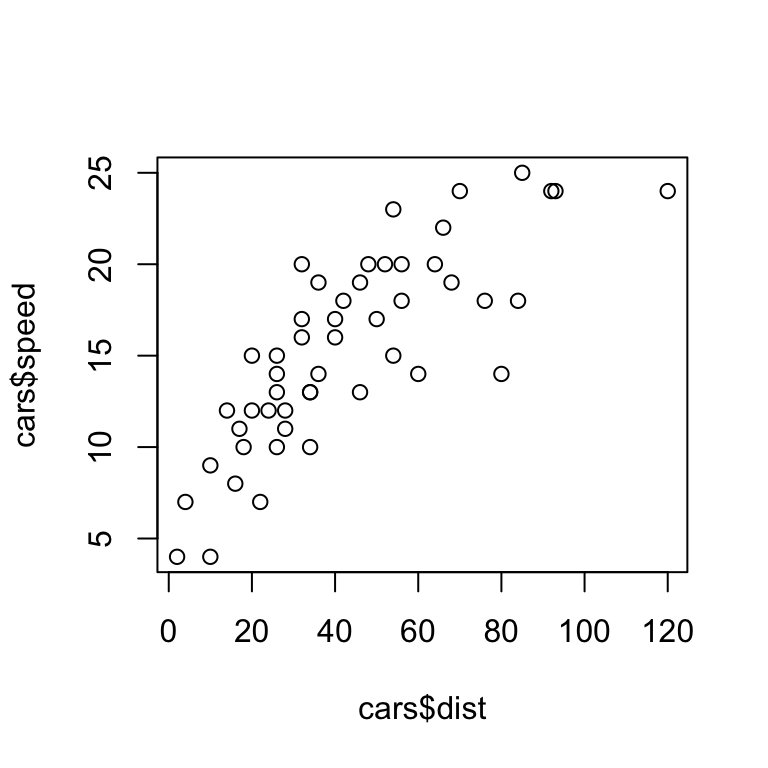## In-class worksheet 1

Jan 21, 2020

Much of the work in this class will be done via R Markdown documents. R Markdown documents are documents that combine text, R code, and R output, including figures. They are a great way to produce self-contained and documented statistical analyses.

In this first worksheet, you will learn how to do some basic markdown editing. After you have made a change to the document, press “Knit HTML” in R Studio and see what kind of a result you get.

Edit only below this line.

## 1. Basic Markdown editing

Try out basic R Markdown features, as described here. Write some text that is bold, and some that is in italics. Make a numbered list and a bulleted list. Make a nested list. Try the block-quote feature.

This text is bold.

This text is in italics.

A numbered list:

1. Item 1
2. Item 2
3. Item 3

A bulleted list:

• Item 1
• Item 2
• Item 3

A nested list:

1. Item 1
• Item 1.1. Note that 4 spaces are required for the nesting to work properly.
• Item 1.2
2. Item 2

Block quote:

“If we knew what it was we were doing, it would not be called research, would it?” — Albert Einstein

## 2. Embedding R code

R code embedded in R chunks will be executed and the output will be shown.

x <- 5
y <- 7
z <- x * y
z
##  35

Play around with some basic R code. E.g., take the built-in data set cars, which lists speed and stopping distance for cars from the 1920. Plot speed vs. distance, and/or perform a correlation analysis. Then write a few sentences describing what you see.

Plot of speed vs. distance:

plot(cars$dist, cars$speed)Correlation analysis:

cor.test(cars$dist, cars$speed)
##
##  Pearson's product-moment correlation
##
## data:  cars$dist and cars$speed
## t = 9.464, df = 48, p-value = 1.49e-12
## alternative hypothesis: true correlation is not equal to 0
## 95 percent confidence interval:
##  0.6816422 0.8862036
## sample estimates:
##       cor
## 0.8068949

There is a strong positive correlations. Cars with higher speed have longer stopping distances.

## 3. If this was easy

If this was easy, use Google to find out how to type-set mathematical formulas inside of R markdown.

Mathematical equations are described here.

For example, the geometric series:

$\sum_{k=0}^\infty r^k = \frac{1}{1-r}$

(Note that this only works if you are online, because a math rendering engine is downloaded on the fly.)